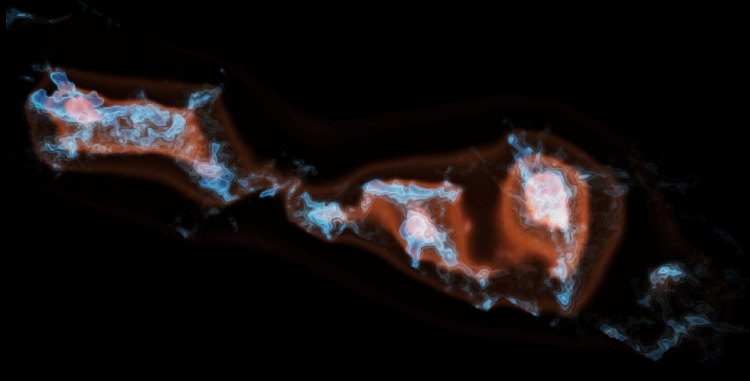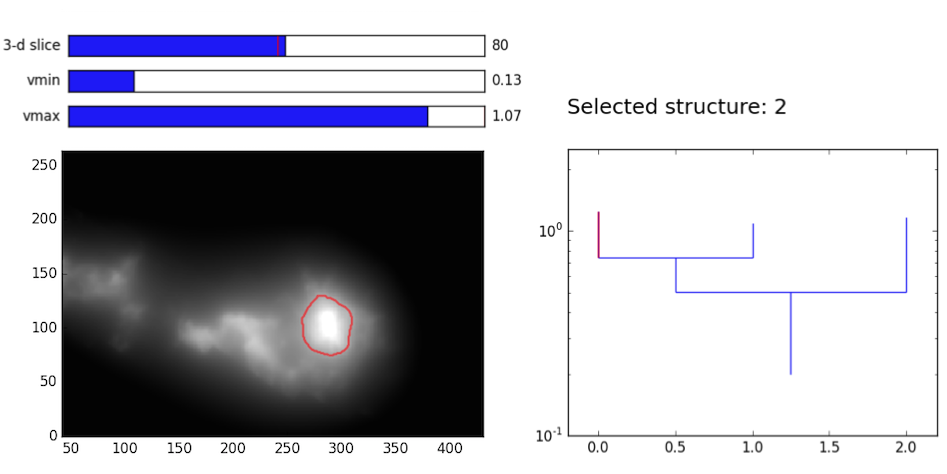# G-virial

Gravity-based structure analysis of molecular clouds

View the Project on GitHub gxli/G-virial

## General concept

Gravity plays important roles in astrophysical conditions. In observations, gas can be observed in Position-Position-Velocity (PPV) space. The G-virial method synthesises the position and velocity informaiton of the gas, and provides a global picture of gravity.

The input of the method is a distrubtion of mass in the PPV space, which can be obtained by mapping the molecular gas with radio telescopes. The output of the method is a map of the importance of gravity in the PPV space (G-virial map).

A movie can be found here .A 3D rendering of the Perseus moleclar cloud. The blue contours stand for gas traced by the 13CO(1-0) emission, and the red contours stand for the G-virial. Created with yt .

## How it works

The G-virial map is derived based on a map of mass distribution. Given a mass distribution

$$\mathrm m(x,y,v)=\rho(x,y,v)\;{\rm d}x\; {\rm d} y \;{\rm d}v\;,$$
The G-virial is defined as
$$\mathrm \alpha (x,y,v)= \int \frac{G \;\rho(x',y',v')}{((x-x')^2+(y-y')^2)^{1/2}} \frac{1}{(v-v')^2 + c_0 ^2}\;{\rm d}x' {\rm d}y' {\rm d}v'\;.$$
The G-virial map is computed according to this definition.

## Getting started

Simply try it out.

1. Download a CO data cube. The axes have to be arranged as (v, y, x).

2. Run the program

python compute_gvirial.py file_in.fits file_out.fits d c_0 npad_fft

where file_in is the input fits file, file_out is the output file, d is the distance of the object measured in pc, c_0 and npad_fft are parameters. Usually, c_0 = 1e5 and npad_fft = 3.

Another way of using the program is to call it as a function in python. Please find more details in the documentation.

The output is a data cube which have the same dimensions as the input. The values in the output represent the importance of gravity across the PPV space. It can be visualized and analyzed with many standard tools, such as the Dendrogram .Visualzing the G-virial map of the Perseus molecular cloud with the Dendrogram program.

## Reference

The G-virial method is introduced in this paper: G-virial: A Gravity-based Method to Quantify the Structure of Molecular Clouds.